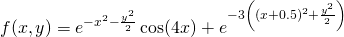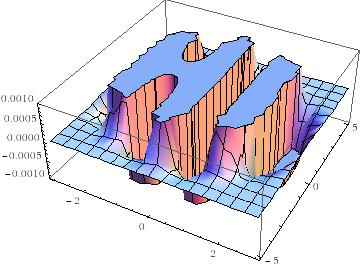## Secret messages hidden inside equations

October 1st, 2007 | Categories: general math, math software, mathematica, matlab | Tags:

At the moment I am writing an introductory Mathematica course and was recently looking for inspiration for potential exercises. One website I came across (I have lost the link unfortunately) suggested that you get something interesting looking if you plot the following equation over the region -3<x<3, -5<y<5. It also suggested that you should only plot the z values in the range 0<z<0.001.Suitably intrigued, I issued the required Mathematica commands and got the plot below which spoke to me in a way that no equation ever has before.So now I have a question – What other messages could one find hidden inside equations like this? For example, is it possible to generate a three letter word with a relatively simple equation such as the one above? Of course if you were allowed to use very complex equations (and make use of Fourier transforms maybe) then I guess you could spell out whatever you choose but that’s no fun.

If anyone finds other such messages in simple(ish) equations then please let me know.

1. Like it! I suppose it’s a new take on “Hello World” for the advanced user!

2. have you plotted its derivative?

3. It’s like a really advanced version of writing “hello” on a digital calculator.

*goes and dusts off his TI-83*

4. Here’s a really weird one – I haven’t tried it myself, but it’s almost unbelievable:
http://www.kirchersociety.org/blog/2007/02/02/tuppers-self-referential-formula/
If I’m reading right, the formula actually plots itself. Hope you like.

5. Math is behind everything!
I love it!

6. are you still intrested?? let me khow i have some very very cool stuff

7. Yep, I’m still interested :)

8. EXQUISITE ! …… that f(x,y) looks more like a function straight our of ‘Potential Theory’, then again I guess it is more aesthetic ! :-)

I guess that it should be possible to do on MATLAB.

9. Can we make a ‘HELLO’ …. ? …. ;) (well…. this may be the start to a new encryption method – via MATLAB ….. LOL ! )

10. Hi,

you can check this post in xamuel.com (the blog of a friend, not mine) where he discusses parametric plots of words:

http://www.xamuel.com/graphs-of-implicit-equations/

You have to scroll down a little first.

Cheers,

Ruben

11. It is definitely an interesting thought towards the realm of steganography :) Not quite encryption/cryptography, but very interesting.

I am also interested in using infinite (transcendental) sequences as keys or for hiding messages if given offsets (similar to knowing the x,y,z ranges to plot).

12. Handcrafted in 12 minutes:

e^(-x^2-100y^2)
+e^(-100*(x-1)^2-y^2/2)
+e^(-100*(x+1)^2-y^2/2)

+e^(-(x-5)^2-100*(y-1)^2)
+e^(-(x-5)^2-100y^2)
+e^(-(x-5)^2-100*(y+1)^2)
+e^(-100*((x-4.5)+1)^2-y^2/2)

+e^(-100*((x-8.5)+1)^2-y^2/2)
+e^(-(x-9)^2-100*(y+1)^2)

+e^(-100*((x-12.5)+1)^2-y^2/2)
+e^(-(x-13)^2-100*(y+1)^2)

+e^(-100*((x-17)+1)^2-y^2/3)
+e^(-(x-18)^2-100*(y+1)^2)
+e^(-(x-18)^2-100*(y-1)^2)
+e^(-100*((x-19)+1)^2-y^2/3)

I leave the world as an excercise

13. the 18s should be 17s

14. I thinkt this one also produces an interesting graph: (2x^2+y^2+z^2-1)^3-x^2z^3/10-y^2z^3=0
[source: http://www.mathematische-basteleien.de/heart.htm%5D

15. this may be salutary for students because it illustrates:
1) moving functions around
2) squeezing functions
4) (non-normalized) variants of the normal distribution

PS: the 18s should be 17s

16. and here is the idea behind it:

| | |– | | |–|
|-| |– | | | |
| | |– |– |– |–|

17. Here you can try out the 3d functions:

http://www.livephysics.com/ptools/online-3d-function-grapher.php?ymin=-3&xmin=-3&zmin=0&ymax=3&xmax=10&zmax=1&f=e^%28-x^2-100*y^2%29%2Be^%28-100*%28x-1%29^2-y^2%2F2%29%2Be^%28-100*%28x%2B1%29^2-y^2%2F2%29

Increase grid size!
For easier viewing paste each letter individually.

18. This site is useful as well:

http://fooplot.com/index3d.php

19. An inequality rather than an equation but Tupper’s self-referential formula is pretty impressive http://mathworld.wolfram.com/TuppersSelf-ReferentialFormula.html

20. According to the mathworld page you linked to ‘The formula itself is a general purpose method of decoding a bitmap stored in the constant k, so it could actually be used to draw any other image’.

So, the image is actually contained in the number

4858450636189713423582095962494202044581400587983244549483093085061934704708809928450644769865524364849997247024915119110411605739177407
8569197543265718554420572104457358836818298237541396343382251994521916512843483329051311931999535024137587652392648746133949068701305622
9581321948111368533953556529085002387509285689269455597428154638651073004910672305893358605254409666435126534936364395712556569593681518
4334857605266940161251266951421550539554519153785457525756590740540157929001765967965480064427829131488548259914721248506352686630476300

and not in the formula. So, it’s cool but not VERY cool IMHO :)

21. simplify -2i+u>-5u